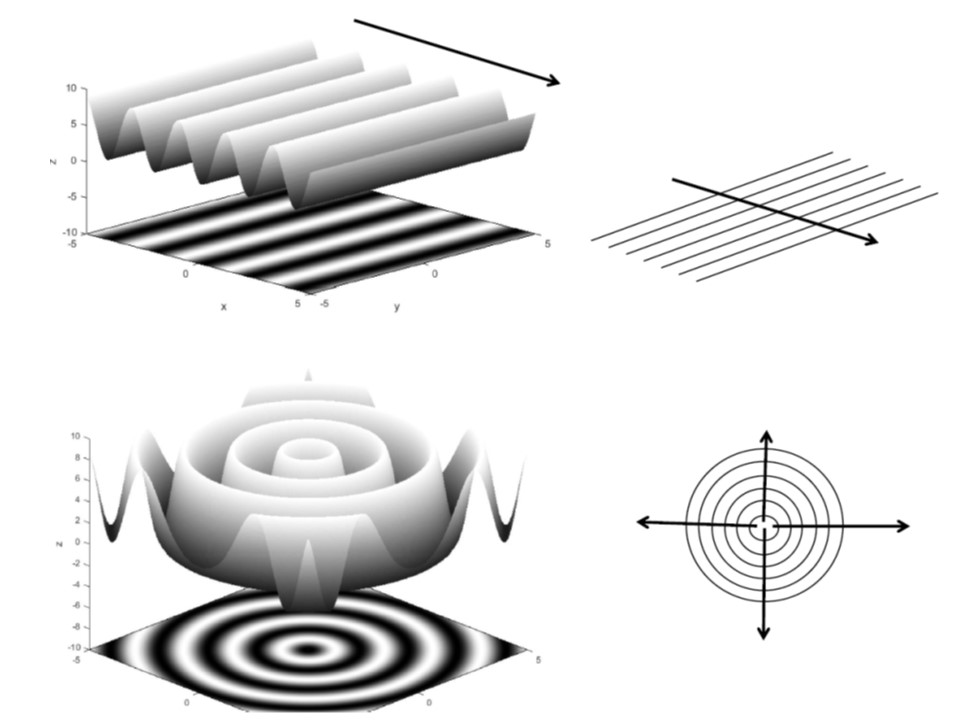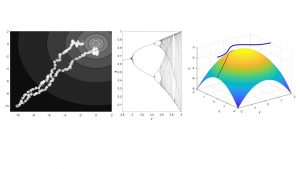# Mathematical Methods in Life Science

The aim of this course is to repeat basic math known from the last year of highschool and to teach an intuitive way to understand math and how to apply it for modeling with simple examples (biased random walk of E-Coli, dynamics of simple gene regulation, growth models, gradient descent, approximation methods). This course also prepares for statistics and programming.

Topics:

▪ derivatives (finite differences, gradient, divergence, Taylor – series, error estimation, simple differential equations, fixed points)

▪ integrals

▪ linear algebra (vectors and matrices, dot product, matrix product, rotation matrices)

▪ basic statistics (probability, normal, Poisson and binomial distribution, mean, variance, covariance and correlation, entropy)

▪ complex numbers